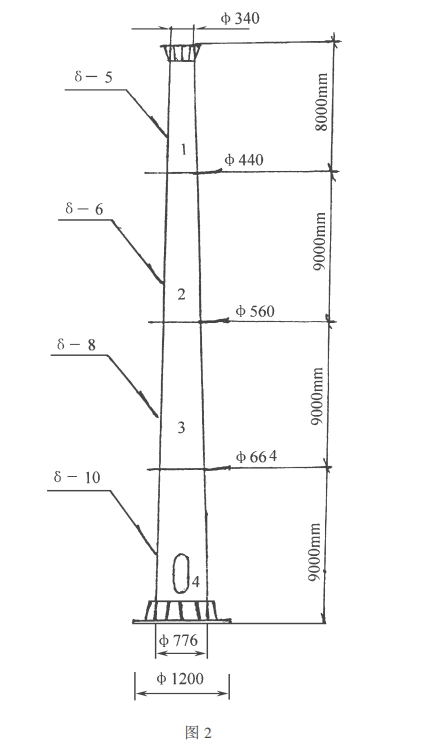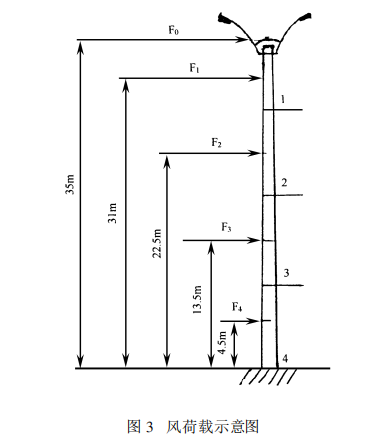0514-84242547# 35米高杆灯的最新设计计算方法1、高杆灯的静力学特征及计算

( 1)基本风压 k0 ,设计风速: V0= 36m /s k0 = V20 1600 = 362 1600 = 0. 81 kN /m2

( 2)实际风压 kRh kRh = Uz _ z _ s ( Ak0 ) 式中: Uz— 风振系数。由于大于 30m高度的建筑物属 于高层建筑 ,而同一高层建筑的不同层次的风振系数 图 2 Uz 的值不同 ,所以 Uzi= 1+ Hi H XV_*z 。其中 H为建筑物 标高 ( 35m); Hi为各层次高度 ,此处分四个层次;X为 脉动增大系数 ,查有关表得;V为脉动影响系数 , B类 地区为 0. 53;k0= 0. 81kN /m2层次结构分四层 , B 类 地区情况下为 1. 28; _ z 为风压高度系数 ,查表得。B 类地形 40m高度以下的建筑层次分别为 35m、 30m、 20m、 10m时 ,_ z 分别取 1. 44、 1. 42、 1. 25、 1. 00; _ s为 体形系数 ,整基高杆灯杆体为圆台形、受风面圆弧 ,_ s 取 0. 8; A为地形地理系数取 1. 2; 各层次的风振系数 Uzi ,计算可得:

Uz1 = 1+ 3535 1. 28× 0. 53 1. 44 = 1. 471; Uz2 = 1+ 3035 1. 28× 0. 53 1. 42 = 1. 478; Uz3 = 1+ 2035 1. 28× 0. 53 1. 25 = 1. 387; Uz4 = 1+ 1035 1. 28× 0. 53 1. 00 = 1. 194 (注: 因灯盘和第一节灯杆的标高基本上可看作 同一高度 ,所以这里稍粗略地分四个层次计算 Uzi 值。)上式 Uzi值分别代入 kRh= Uz _ z _ s ( Ak0 ) ,得: kR0= 1. 471× 1. 44× 0. 8× 1. 2× 0. 81 = 1. 647kN /m2 (灯盘 ) 且: kR1= kR0= 1. 647kN /m2 kR2= 1. 478× 1. 42× 0. 8× 1. 2× 0. 81 = 1. 632kN /m2 kR3= 1. 387× 1. 25× 0. 8× 1. 2× 0. 81 = 1. 348kN /m2 kR4= 1. 194× 1. 00× 0. 8× 1. 2× 0. 81 = 0. 928kN /m2

( 3)各层次的风荷载作用力 F F = kRh S 式中: S— 受风面积 , m2 ∴F0 = kR 0 S0 = 1. 647× 5. 2+ 0. 81 2 × 1. 2× 0. 4 = 2. 376kN (注: 因灯盘的漏空系数为 0. 6,所以实际受风面 为 S0的 0. 4倍 ) F1= kR1 S1 = 1. 647× 0. 35+ 0. 44 2 × 7. 5 = 4. 88kN (注: 灯盘挂杆顶时遮去杆梢上部约 0. 5m,所以 第一灯杆受风高度为 7. 5m) F2 = kR2 S2 = 1. 632× 0. 44+ 0. 56 2 × 9 = 7. 344kN F3 = kR3 S3 = 1. 348× 0. 56+ 0. 664 2 × 9 = 7. 425kN F4 = kR4 S4 = 0. 928× 0. 664+ 0. 776 2 × 9 = 6. 013kN

( 4)高杆灯风荷载扰度。经有关计算 ,此风荷载扰 度 f A= 92cm,扰度占总高度的百分比为: Z= f AH = 92 3500 = 2. 6% 。

( 5)高杆灯各节杆根部的风弯矩 Mi。风荷载计算结合图 3所示 . M1= F0 h1 + F1 12 h1 = 2. 376× 8+ 4. 88× 4 = 38. 528kN m M2= F0 h2 + F1 (h2 - 12 h1 ) + F2 12h1, 2 = 2. 376× 17+ 4. 88× 12. 5+ 7. 344× 4. 5 = 134. 44kN m M3= F0 h3 + F1 (h1, 3 + 12 h1 ) + F2 (h2, 3 + 12 h1, 2 ) + F3 12h2, 3 = 2. 376× 26+ 4. 88× 22+ 7. 344× 13. 5+ 7. 425× 4. 5 = 301. 693 kNm M4= 2. 376× 35+ 4. 88× 31+ 7. 344× 22. 5+ 7. 425× 13. 5+ 6. 013× 4. 5 = 526. 98kN m (注: 同样情况下的正 12棱台形杆体 ,杆根处的风 荷载在尚不包括扰度时的附加弯矩就达 754. 9kN m。 因为圆台形杆体的体形系数 _ s 是 0. 8,而正 12边棱台 形的体形系数是 1. 146— 据同济大学《高层建筑结构设计与施工》一书 P48的公式: 正 n 边形的体形系数 _ s = 0. 8+ 1. 2n , n 为边数 ,得出: _ s= 0. 8+ 1. 212= 1. 146。这说明在同等条件下 ,正 12边形杆灯的风弯矩 是圆台形杆体的 1. 146 /0. 8= 1. 4325倍 ,所以海岛上 灯塔 ,城市的自来水塔及我国许多地区引进的国外高 杆灯如沪宁公路上的等等 ,它们都选择圆台形 ,而不 采用正 12边形。再则从杆体上相同截面积的情况看 , 圆形的刚度比正 12边形的刚度大得多 ,所以 ,导弹、火 箭为圆形而不采用多边形 ,这种情况请高杆灯领域的 同志们引起足够重视。)

( 6)高杆灯受风荷载作用时 ,所引起的扰度 f A而 带来的附加弯矩: ΔMi = G0 f A + Gn 0. 5 f A G0— 组装好的高杆灯的灯盘重量; Gn— 整个杆体部分 的重量; f A— 扰度 92cm(计算略 )。 整个 35m高杆灯的自重情况: 灯盘及组装的 12 只一体化灯具共重 460kg ,杆稍三角支架及罩 150kg , G0= 460+ 150= 610kg= 5. 978kN。杆体重量: 第一灯 杆 475kg加杆梢衬托部分 50kg小计 525kg,第二节灯 杆 658kg,第三节灯杆 1 072kg ,第四节灯杆 2 436kg , 三节衬套 100kg ,卷扬机组 100kg ,钢索和牵引件、电 缆 100kg,杆根底板及支撑板 150kg ,合计 Gn= 525+ 658+ 1072+ 2436+ 100+ 100+ 100+ 150= 5 141kg = 50. 382kN。高杆灯重量 ΢G= 56. 36kN。 ∴ΔM4= 5. 978× 0. 92+ 50. 382× 0. 5× 0. 92 = 28. 68kNm EM4 = M4+ ΔM4 = 526. 98+ 28. 68 = 555. 66kNm ΔM4 /EM4= 28. 68 /555. 66 = 5. 16% ∴EM1= M1+ ΔM1 = 38. 528× 1. 0516 = 40. 52kNm EM2 = M2+ ΔM2 = 134. 44× 1. 0516 = 141. 38kNm EM3 = M3+ ΔM3 = 301. 693× 1. 0516 = 317. 26kNm ( 7)各节杆体杆根部的应力 W的验算。 W= EMW 式中: W— 灯杆杆根部截面抵抗矩 W = 0. 0982× D4 - d4 D ; 式中: D— 杆根外径 , d— 杆根内径。

①第一节杆根处的 W1和钢材使用中的安全系数 T1 (其壁厚 5mm,杆根外径 44cm,内径 43cm) ∴W1 = 0. 0982× 444 - 434 44 = 734. 93cm3 整体四节灯杆的钢材型号为 Q235A型、屈服点 为 23 500N /cm2 , 即 235M Pa,设计计算时允许采用值 为 20 600N /cm2即 206M Pa。

②第二节灯杆杆根处的应力 W2和钢材使用的安 全 系 数 T2 (其 壁 厚 6mm, 杆 根 外 径 56cm, 内 径 54. 8cm)。 ∴W2 = 0. 0982× 564 - 54. 84 56 = 1 431. 35cm3 杆 根 的 应 力 W2 = EM2 W2 = 14 138 000 1 431. 35 = 9 877. 39N /cm2 安全系数 T2= 20 600 /9 877. 39= 2. 09> 1. 64

③第三节灯杆杆根处的应力 W3 ,钢材使用的安 全系数 T3 (杆体壁厚 8mm,杆根外径 66. 4cm,内径 64. 8cm)。 W3 = 0. 0982× 66. 44 - 64. 84 66. 4 = 2 672. 38cm2 杆 根 的 应 力 W3 = EM3 W3 = 31 726 000 2 672. 38 = 11 871. 8 N /cm2 安全系数 T3= 20 600 /11 871. 8= 1. 73> 1. 64

④第四节灯杆杆根处的应力 W4 ,钢材使用的安 全系数 T4 (杆体壁厚 10mm,杆根外径 77. 6cm,内径 75. 6cm)。 W4 = 0. 0982× 77. 64 - 75. 64 77. 6 = 4 550. 94cm3 杆 根 的 应 力 W4 = EM4 W4 = 55 566 000 4 550. 9 = 12 209. 8N /cm2 安全系数 T4= 20 600 /12 209. 8= 1. 69> 1. 64。 以上可得出 ,此高杆灯地上部分设计符合要求。

2、高杆灯地基承载力的验算 高杆灯地基的基础设计草图见图 4。( 1)基础的总重量 GJ GJ = [( 4. 5× 4. 5× 1 - 1. 22 × 3. 14× 2. 6) × 2. 4+ ( 4. 5× 4. 5× 1 - 1. 22× 3. 14× 2. 6) × 1. 8]× 9. 8 = 1 022. 4kN 式中: 2. 4— 钢筋结构后 C20砼浇的密度; 1. 8— 掩埋土 层的密度;× 9. 8— 重量 kg化为 kN

( 2)基础地面处 C20砼浇层的抵抗矩 W W = ( 2 /12) B3 = ( 2 /12) × 4. 53 = 10. 74m3 式中: B— 边长 ,取 4. 5m.

( 3)标准地基的承载值 90kN /m 的设计值按 f = 1. 1fk计算 f = 1. 1× 90= 99kN /m2

( 4)基础的平均压强按 P= EG+ GJ A 计算 , A— 基础底 面积∴P= 56. 36+ 1 022. 4 4. 52 = 53. 27kN /m2 < 90kN /m2 (标 准承载 )

( 5)基础边缘有可能产生的最大压强 Pmax Pmax = P + EM4 W4 (原公式: Pmax = P + Me+ EM4 W ) 其中: M e— 高杆灯杆体部分重心不在基础中心的偏 心弯矩 ,然此设计中重心皆在同一铅直线上 ,所以偏 心弯矩 M e= 0,即 Pmax = P + EM4 W = 53. 27+ 555. 66 10. 74 = 105kN /m2 ( 6)根据 GB J7— 89第 5. 1. 1各建筑地基基础设计 规范 ,应按基础平均压强 P≤ f , Pmax≤ 1. 2f 验算。 ∵P = 53. 27kN /m2 < f ( 99kN /m2 ) 又: Pmax = 105kN /m2 < 1. 2× 99 所以上述 35m高杆灯的基础设计是完全符合规范的。